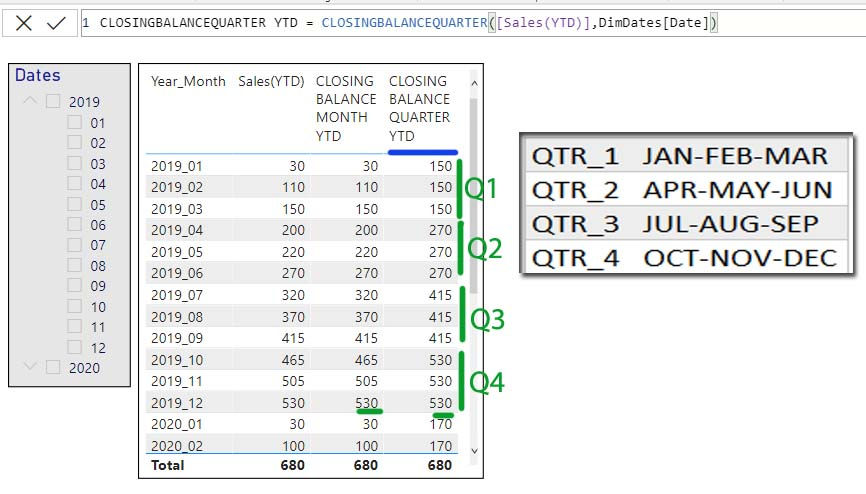top of page
Search
• Marek Vavrovic

# Time intelligence functions, part 01

## Notes

In order to use any time intelligence calculation, you need a well-formed date table. The Date table must satisfy the following requirements:

• All dates need to be present for the years required. The Date table must always start on January 1 and end on December 31, including all the days in this range. If the report only references fiscal years, then the date table must include all the dates from the first to the last day of a fiscal year. For example, if the fiscal year 2008 starts on July 1, 2007, then the Date table must include all the days from July 1, 2007 to June 30, 2008.

• There needs to be a column with a DateTime or Date data type containing unique values. This column is usually called Date. Even though the Date column is often used to define relationships with other tables, this is not required. Still, the Date column must contain unique values and should be referenced by the Mark as Date Table feature. In case the column also contains a time part, no time should be used – for example, the time should always be 12:00 am.

• The Date table must be marked as a date table in the model, in case the relationship between the Date table and any other table is not based on the Date.

CLOSINGBALANCEMONTH(<expression>,<dates>[,<filter>])

Evaluates the expression at the last date of the month in the current context.

Function corresponds to ENDOFMONTH()

CLOSINGBALANCEQUARTER(<expression>,<dates>[,<filter>])

Evaluates the expression at the last date of the quarter in the current context.

Function corresponds to ENDOFQUARTER()

CLOSINGBALANCEYEAR(<expression>,<dates>[,<filter>][,<year_end_date>])

Evaluates the expression at the last date of the year in the current context.

Function corresponds to ENDOFYEAR

OPENINGBALANCEMONTH(<expression>,<dates>[,<filter>])

Evaluates the expression at the first date of the month in the current context.

Function corresponds to PREVIOUSDAY(STARTOFQUARTER(<Dates> ))

OPENINGBALANCEQUARTER(<expression>,<dates>[,<filter>])

Evaluates the expression at the first date of the quarter, in the current context.

Function corresponds to PREVIOUSDAY(STARTOFQUARTER(<Dates> ))

OPENINGBALANCEYEAR(<expression>,<dates>[,<filter>][,<year_end_date>])

Evaluates the expression at the first date of the year in the current context.

similar to: PREVIOUSDAY(STARTOFYEAR(<Dates> [,<YearEndDate>]))

Data and Data Model

FactSales table contains 2-3 records per month of the daily sales during two years(2019-2020)EXAMPLE 1

## CLOSINGBALANCEMONTH

CLOSINGBALANCEMONTH(<expression>,<dates>[,<filter>])

Evaluates the expression at the last date of the month in the current context.

Sales column contains daily sales, Sales(YTD) calculates cumulative total. CLOSINGBALANCEMONTH() works with these cumulative data and at the last date of the month returns the final number for each row of that particular month.CLOSINGBALANCEMONTH() correspond to ENDOFMONTH() function

CALCULATE ( <Expression>, ENDOFMONTH ( <Dates> ) [, <Filter>])

Sales(YTD) = CALCULATE(SUM(FactSales[Sales]),DATESYTD(DimDates[Date]))CLOSINGBALANCEMONTH()

using this function just with months## CLOSINGBALANCEQUARTER

CLOSINGBALANCEQUARTER(<expression>,<dates>[,<filter>])

Evaluates the expression at the last date of the quarter in the current context.

similar to:

CALCULATE ( <Expression>, ENDOFQUARTER ( <Dates> ) [, <Filter>])

Example: CLOSINGBALANCEQUATER

CLOSINGBALANCEQUARTER YTD = CLOSINGBALANCEQUARTER([Sales(YTD)],DimDates[Date])EXAMPLE:

ENDOFQUATER

ENDOFQUARTER = CALCULATE([Sales(YTD)],ENDOFQUARTER(DimDates[Date]))

Sales(YTD) = CALCULATE(SUM(FactSales[Sales]),DATESYTD(DimDates[Date]))## CLOSINGBALANCEYEAR

this function is similar to ENDOFYEAR()## OPENINGBALANCEMONTH

function is similar to:

CALCULATE (<Expression>,

PREVIOUSDAY ( STARTOFMONTH ( <Dates> ) )

[, <Filter>])## OPENINGBALANCEQUARTER

similar to:

CALCULATE (<Expression>,

PREVIOUSDAY ( STARTOFQUATER ( <Dates> ) )

[, <Filter>])another function, that works with quaters is NEXTQUATER()## OPENINGBALANCEYEAR

this function is similar to:

CALCULATE (

<Expression>,

PREVIOUSDAY ( STARTOFYEAR ( <Dates> [, <YearEndDate>] ) )

[, <Filter>]

)